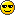# Ch 14.1 Help##Ch 14.1 Help

1) A gas expands at a constant pressure of 2.987 atm its volume from 9.813 liter to 19.99 liter. What is the work done by the gas (see sheet 7; include the correct sign) ?
How to solve:

2) 32.43 cal heat are added to a gas which does 211.2 J work by expanding. What is the change in internal energy of the gas (see sheet? Indicate with a positive (negative) sign whether the internal energy increases (decreases).
How to solve:

3) 1.592 moles of a gas at room temperature (20 oC) expand quickly ("adiabatically") at an average pressure of 2.766 atm increasing its volume by 257.8 cm3. What is the final temperature in oC (see sheet 7,8,11') ? (NOTE! The change in volume given, not the volume itself. Thus you cannot use the gas equation to get the number of molecules.) Indicate with a positive (negative) sign whether the temperature decreases (increases).
How to solve:

4) The internal energy of a gas is quickly ("adiabatically") increased by 387.6 J at an average pressure of 4.257 atm. What is the change in volume (see sheet 7,? Indicate with a negative (positive) sign whether the gas is compressed (expands).
How to solve:

##Question 2 and 4

Anyone know how to do 2 and 4?

angelbab
Guest

##Questions 1&2

Question 1

W = P*Change in Volume
Change in volume = 10.177 (I just subtracted the two to find the difference) - keep in mind this is still in Liters though so divide that by 1000 to put into m^3 = .010177
Then I did # in atm * 101300 to put into pascals
2.987 * 101300 = 302583.1
Then i did 302583.1 * .010177 = 3079.388 answer is positive

Question 2
First, convert the calories to joules by multiplying by 4.187 since there are 4.187 J in 1 cal
32.43 cal * 4.187 = 135.78441
Then to find the difference in internal energy, subtract the other number you just calculated from the other number already given in joules
211.2 J - 135.78441 = 75.41559

Kathleen
Guest

##Question 4

Question 4

Q = DeltaU + W
They say that U(internal energy) = 387.6
W = P*Change in Volume

So you can plug in and do Q=U + P*Change in volume
It says on sheet 8 that for adiabatic Q=0 so do
0 = 387.6 + 431234.1*Change in volume
(I got the 431234.1 by multiplying the pressure in atm which was 4.257*101300)
You solve and get Change in Volume = -.0008988157

Kathleen
Guest

##Q3

Anyone know how to do question 3?

meg
Guest

##Re: Ch 14.1 Help

I'm still working on question 3 but I assume you have to plug in both the equations into Q

So, where Q = DeltaU + W
You would do Q = (3/2)(n)(k)(T) + P(Change in Volume)
Possibly with a Tf-Ti where the T is and solve for Tf but that doesn't seem to be working for me, I converted all the values but I'm not getting anywhere yet. I'll let you know.

Kathleen
Guest

##Question 3

Ive been trying to do three as well I cant figure it out anyone have an idea?

hwilson
Guest

##Question 3

Question 3
Delta U = -W
W = P*Change in Volume
P = 2.766 atm * 101300 = 280195.8 Pa
V = 257.8 cm^3 / 1000000 = .0002578 m^3
W = 280195.8 * .0002578 = 72.23447724

Delta U = (3/2)*N*k*(Tf-Ti)
N = 1.592 * (6.022e23) = 9.587024e23
K = constant which is 1.38e-23
Tf = final temperature, what you are looking to find
Ti = 20 degrees celsius

(3/2)*(9.587024e23)*(1.38e-23)*(Tf-20) = -72.234477
19.845139*Tf - 396.90279 = -72.234477
19.845139*Tf = 324.6683166
Tf = 16.36

sometimes the second decimal will be off, but as long as you don't go past that you should be fine. I rounded to the third in my actual answer on maple TA and that worked so...idk

Kathleen
Guest

##Re: Ch 14.1 Help

How did you get 396.90279?

##in response to guest

(3/2)*(9.587024e23)*(1.38e-23)*(Tf-20) = -72.234477
19.845139*Tf - 396.90279 = -72.234477
19.845139*Tf = 324.6683166

~~~~~~~~~~~~~
First, i multiplied the (3/2)*(9.587024e23)*(1.38e-23) to get 19.84 then
I factored out with the parentheses so i multiplied the 19.84 by Tf and then by 20 so then I had 19.84Tf - 19.84*(20) = 19.84Tf - 396.90

Kathleen
Guest

##Re: Ch 14.1 Help